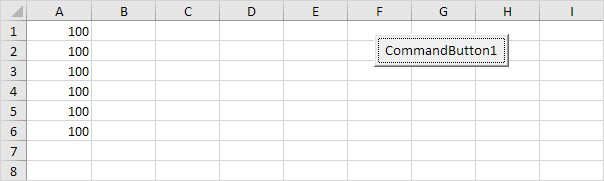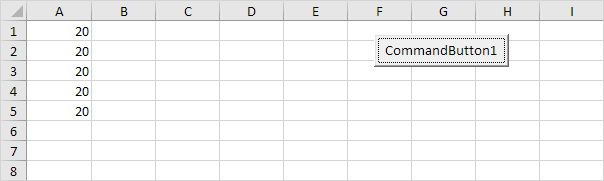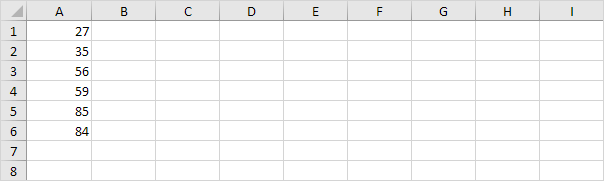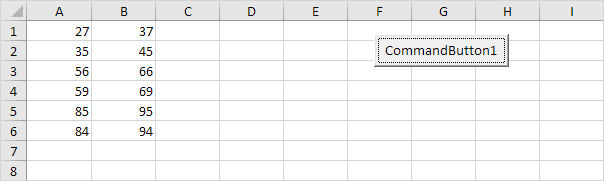# Loop

Looping is one of the most powerful programming techniques. A loop in Excel VBA enables you to loop through a range of cells with just a few codes lines.

### Single Loop

You can use a single loop to loop through a one-dimensional range of cells.

Place a command button on your worksheet and add the following code lines:

Dim i As Integer

For i = 1 To 6
Cells(i, 1).Value = 100
Next i

Result when you click the command button on the sheet:Explanation: The code lines between For and Next will be executed six times. For i = 1, Excel VBA enters the value 100 into the cell at the intersection of row 1 and column 1. When Excel VBA reaches Next i, it increases i with 1 and jumps back to the For statement. For i = 2, Excel VBA enters the value 100 into the cell at the intersection of row 2 and column 1, etc.

Note: it is good practice to always indent (tab) the code between the words For and Next. This makes your code easier to read.

### Double Loop

You can use a double loop to loop through a two-dimensional range of cells.

Place a command button on your worksheet and add the following code lines:

Dim i As Integer, j As Integer

For i = 1 To 6
For j = 1 To 2
Cells(i, j).Value = 100
Next j
Next i

Result when you click the command button on the sheet:Explanation: For i = 1 and j = 1, Excel VBA enters the value 100 into the cell at the intersection of row 1 and column 1. When Excel VBA reaches Next j, it increases j with 1 and jumps back to the For j statement. For i = 1 and j = 2, Excel VBA enters the value 100 into the cell at the intersection of row 1 and column 2. Next, Excel VBA ignores Next j because j only runs from 1 to 2. When Excel VBA reaches Next i, it increases i with 1 and jumps back to the For i statement. For i = 2 and j = 1, Excel VBA enters the value 100 into the cell at the intersection of row 2 and column 1, etc.

### Triple Loop

You can use a triple loop to loop through two-dimensional ranges on multiple Excel worksheets.

Place a command button on your worksheet and add the following code lines:

Dim c As Integer, i As Integer, j As Integer

For c = 1 To 3
For i = 1 To 6
For j = 1 To 2
Worksheets(c).Cells(i, j).Value = 100
Next j
Next i
Next c

Explanation: The only change made compared to the code for the double loop is that we have added one more loop and added Worksheets(c). in front of Cells to get the two-dimensional range on the first sheet for c = 1, the second sheet for c = 2 and the third sheet for c = 3. Download the Excel file to see this result.

### Do While Loop

Besides the For Next loop, there are other loops in Excel VBA. For example, the Do While Loop. Code placed between Do While and Loop will be repeated as long as the part after Do While is true.

1. Place a command button on your worksheet and add the following code lines:

Dim i As Integer
i = 1

Do While i < 6
Cells(i, 1).Value = 20
i = i + 1
Loop

Result when you click the command button on the sheet:Explanation: as long as i is lower than 6, Excel VBA enters the value 20 into the cell at the intersection of row i and column 1 and increments i by 1. In Excel VBA (and in other programming languages), the symbol '=' means becomes. It does not mean equal. So i = i + 1 means i becomes i + 1. In other words: take the present value of i and add 1 to it. For example, if i = 1, i becomes 1 + 1 = 2. As a result, the value 20 will be placed into column A five times (not six because Excel VBA stops when i equals 6).

2. Enter some numbers in column A.3. Place a command button on your worksheet and add the following code lines:

Dim i As Integer
i = 1

Do While Cells(i, 1).Value <> ""
Cells(i, 2).Value = Cells(i, 1).Value + 10
i = i + 1
Loop

Result when you click the command button on the sheet:Explanation: as long as Cells(i, 1).Value is not empty (<> means not equal to), Excel VBA enters the value into the cell at the intersection of row i and column 2, that is 10 higher than the value in the cell at the intersection of row i and column 1. Excel VBA stops when i equals 7 because Cells(7, 1).Value is empty. This is a great way to loop through any number of rows on a worksheet.

Go to Next Chapter: Macro Errors